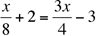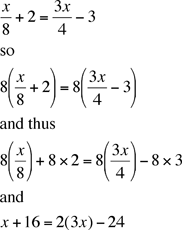SEARCH HOMEMath Central Quandaries & QueriesSubject: Algebra 2 Name: Fabiola Who are you: Student x divided by 8+2= 3x divided by 4-3. need to solve for xFabiola,

I would first clear the fractions. You haveWhat I see from the denominators of 8 and 4 is that if I multiply both sides by 8 the fractions are eliminated.Now solve for x.

PennyMath Central is supported by the University of Regina and The Pacific Institute for the Mathematical Sciences.